# nonlinearTseries Quickstart

#### 2021-05-12

The nonlinearTseries package provides functionality for nonlinear time series analysis. This package permits the computation of the most-used nonlinear statistics/algorithms including generalized correlation dimension, information dimension, largest Lyapunov exponent, sample entropy and Recurrence Quantification Analysis (RQA), among others. Basic routines for surrogate data testing are also included. This vignette provides a brief overview of the most important routines contained in nonlinearTseries.

## Data: Lorenz system

To explore the routines included in nonlinearTseries, we will study the famous Lorenz system. nonlinearTseries offers different routines for simulating the best well-known nonlinear systems:

suppressMessages(library('nonlinearTseries'))
library('plot3D')
# by default, the simulation creates a RGL plot of the system's phase space
lor = lorenz(do.plot = F)
# let's plot the phase space of the simulated lorenz system
scatter3D(lor$x, lor$y, lor$z, main = "Lorenz's system phase space", col = 1, type="o",cex = 0.3)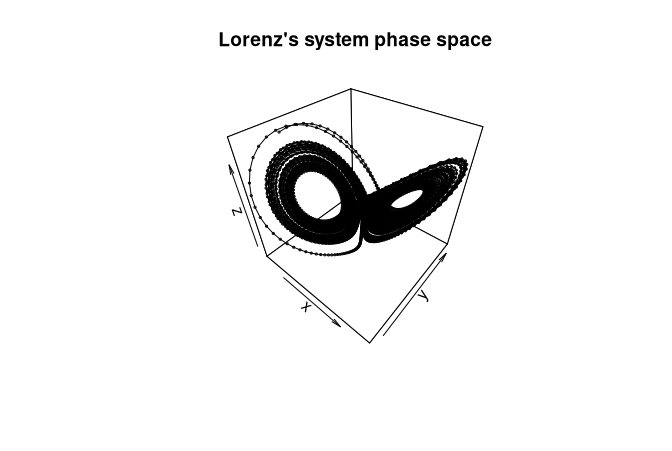It must be noted that the lorenz function returns the simulated components of the system in a list. Future versions of the package will allow to obtain the same simulations as ts objects. A complete list of the available functions for nonlinear systems simulation can be found in the lorenz help page (?lorenz command). ## Taken’s embedding theorem Usually, what we observe in a physical experiment is a single time series and not the complete phase space. For example, let’s assume that we have only measured the $$x$$ component of the Lorenz system. Fortunately, we can still infer the properties of the phase space by constructing a set of vectors whose components are time delayed versions of the $$x$$ signal $$[x(t), x(t+\tau), ..., x(t + m\tau)]$$ (This theoretical result is referred to as the Takens’ embedding theorem). The nonlinearTseries package provides functions for estimating proper values of the embedding dimension $$m$$ and the delay-parameter $$\tau$$. First, the delay-parameter can be estimated by using the autocorrelation function or the average mutual information of the signal. # suppose that we have only measured the x-component of the Lorenz system lor.x = lor$x

old.par = par(mfrow = c(1, 2))
# tau-delay estimation based on the autocorrelation function
tau.acf = timeLag(lor.x, technique = "acf",
lag.max = 100, do.plot = T)
# tau-delay estimation based on the mutual information function
tau.ami = timeLag(lor.x, technique = "ami",
lag.max = 100, do.plot = T)
par(old.par)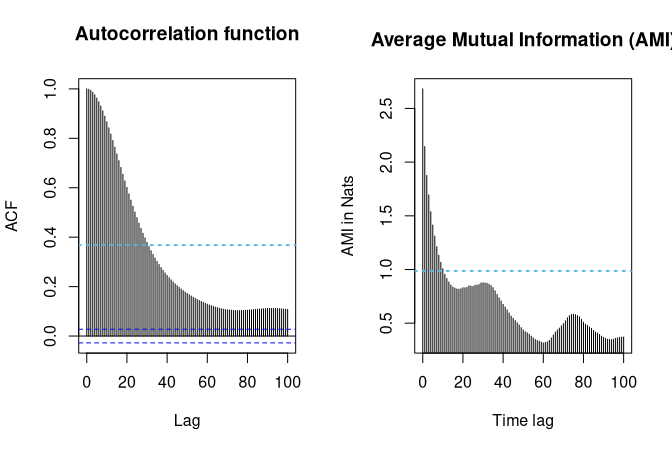Both techniques select a time-lag based on the behavior of the autocorrelation or the average mutual information function. Since the autocorrelation function is a linear statistic we usually obtain more appropriate values with the mutual information technique. Thus, for the remainder of this section, we will use the value obtained with this technique.

Once the time-lag parameter has been estimated, a proper embedding dimension can be computed by using the well-known Cao’s algorithm (see the documentation of the estimateEmbeddingDim function for references):

emb.dim = estimateEmbeddingDim(lor.x, time.lag = tau.ami,
max.embedding.dim = 15)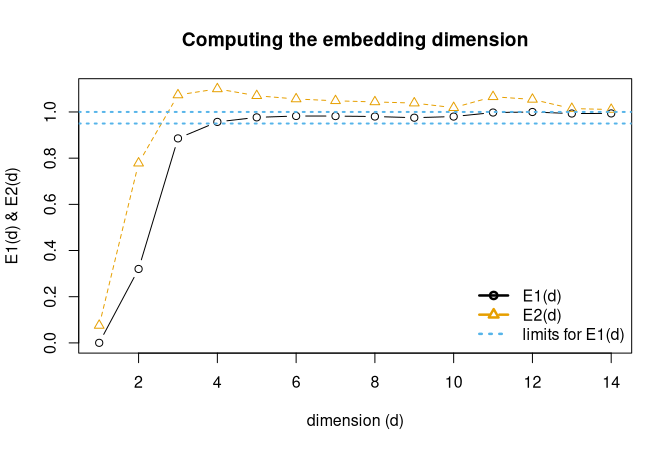When applied to the Lorenz system, the Cao’s algorithm suggests the use of an embedding dimension of 4. The final phase space reconstruction can be obtained using the buildTakens function:

tak = buildTakens(lor.x,embedding.dim = emb.dim, time.lag = tau.ami)
scatter3D(tak[,1], tak[,2], tak[,3],
main = "Lorenz's system reconstructed phase space",
col = 1, type="o",cex = 0.3)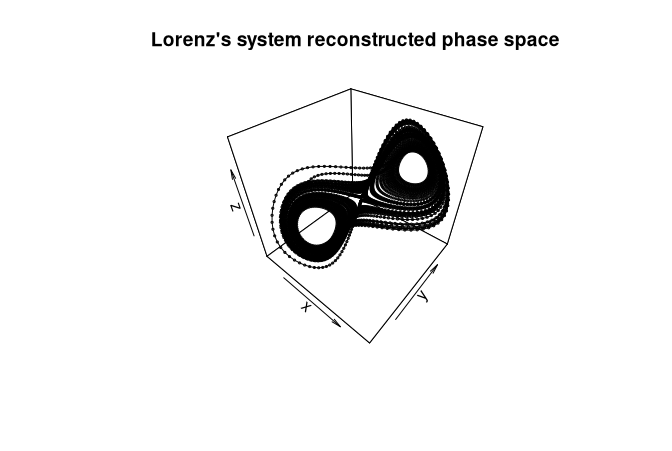Note that the reconstructed and the original phase space, although different, share similar topological features.

## Lyapunov exponent and dimensions

In practical applications, some of the best well-known nonlinear statistics (such as the Lyapunov exponent, the generalized correlation dimensions or the sample entropies) share a similar estimation process. This process could be summarized as follows:

• Perform some heavy computations characterizing either the scaling behavior of the attractor in the phase space (e.g. correlation dimension) or the dynamical evolution of the system in time
(e.g. Lyapunov exponent). These computations are usually repeated for several embedding dimensions.
• The estimation of the nonlinear statistic requires the existence of a small region (in space or time) in which the function computed in the previous step manifests a linear behavior. The slope of this linear region yields the value of the nonlinear statistic. Since the nonlinear statistics in which we are interested in are invariants of the dynamical system, their values should not depend on the embedding dimension used to estimate them (provided that we are using an embedding dimension large enough to reconstruct the phase space). Thus, it is important to check for the existence of this linear region through plots. We should also check that the slope of this region does not depend on the embedding dimension. The plot function can be used with all the objects involved in the computation of these statistics.
• Once the linear-region has been localized, the nonlinear statistic is obtained by performing a linear regression using the estimate function.

In the following sections we illustrate this procedure computing the correlation dimension, the sample entropy and the Lyapunov exponents of the Lorenz system.

### Correlation dimension

The correlation dimension is a technique that measures the fractal dimension of the phase space of a dynamical system. To verify that the estimation of the correlation dimension does not depend on the embedding dimension, we compute the correlation sums (corrDim function) for several embedding dimensions. Once we have checked for the existence of the linear regions in different embedding dimensions, we obtain an estimation of the correlation dimension with the estimate function. This function allows to specify the range in which the linear behavior appears (regression.range parameter) as well as the embedding dimensions to be used for the estimation of the correlation dimension (use.embeddings parameter). The final estimation of the correlation dimension is an average of the slopes obtained for each embedding dimension.

cd = corrDim(lor.x,
min.embedding.dim = emb.dim,
max.embedding.dim = emb.dim + 5,
time.lag = tau.ami,
do.plot=FALSE)
plot(cd)
cd.est = estimate(cd, regression.range=c(0.75,3),
use.embeddings = 5:7)
cat("expected: 2.05  --- estimate: ",cd.est,"\n")
## expected: 2.05  --- estimate:  1.994026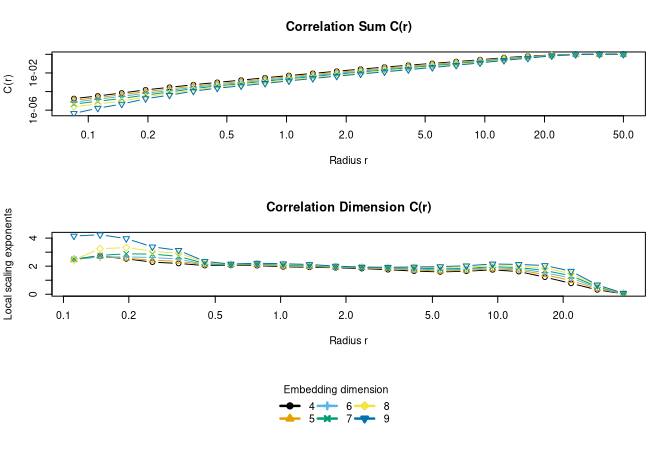The generalized correlation dimensions can also be computed with the corrDim function (by modifying the q parameter). To estimate the information dimension, nonlinearTseries provides the infDim function (see ?infDim for more information).

## Sample entropy

The sample entropy is a technique for measuring the unpredictability of a time series. It is possible to use the correlation sums for obtaining an estimation of the sample entropy of a time series. In this case, the computations should yield a function with a clear plateau. The value of this plateau is an estimation of the sample entropy. The next chunk of code illustrates the procedure for estimating the sample entropy from a previously computed corrDim object.

se = sampleEntropy(cd, do.plot = F)
se.est = estimate(se, do.plot = F,
regression.range = c(8,15))
cat("Sample entropy estimate: ", mean(se.est), "\n")
## Sample entropy estimate:  0.1440437

## Maximum Lyapunov exponent

One of the more important characteristics of a chaotic system is its sensitivity to initial conditions. As a consequence of this sensitivity, close trajectories diverge exponentially fast. The maximum Lyapunov exponent measures the average rate of divergence of close trajectories in the system. The maxLyapunov function can be used for computing this divergence through time. To define what is a close trajectory we make use of the radius parameter. After the computation of the divergence rates we can get an estimate of the maximum Lyapunov exponent by performing a linear regression (estimate function), just as we did with the correlation dimension.

# get the sampling period of the lorenz simulation
# computing the differences of time (all differences should be equal)
sampling.period = diff(lor\$time)
ml = maxLyapunov(lor.x,
sampling.period=0.01,
min.embedding.dim = emb.dim,
max.embedding.dim = emb.dim + 3,
time.lag = tau.ami,
max.time.steps=1000,
do.plot=FALSE)
plot(ml,type="l", xlim = c(0,8))
ml.est = estimate(ml, regression.range = c(0,3),
do.plot = T,type="l")
cat("expected: 0.906  --- estimate: ", ml.est,"\n")
## expected: 0.906  --- estimate:  1.005747

## Surrogate data testing

Although we have postponed its discussion until the end of this vignette, the first step before studying a system using nonlinear analysis techniques should be checking that the data shows indeed some degree of nonlinearity.

The preferred method for nonlinearity-test in literature is surrogate data testing. In surrogate data testing, a statistic $$\mu$$ quantifying some nonlinear feature of the data is computed and compared with the resulting values for an ensemble of comparable linear processes.

nonlinearTseries includes basic functionality for surrogate data testing. The next example performs surrogate data testing by measuring the time asymmetry of the data and the surrogates (since linear stochastic processes are symmetric under time reversal, a deviation from the distribution of the surrogates would be a strong sign of nonlinearity). From the resulting figure, it is clear that our time series shows some degree of nonlinearity.

st = surrogateTest(lor.x,significance = 0.05,one.sided = F,
FUN = timeAsymmetry, do.plot=F)
## Computing statistics
## Null Hypothesis: Data comes from a linear stochastic process
## Reject Null hypothesis:
##      Original data's stat is significant larger than surrogates' stats
plot(st)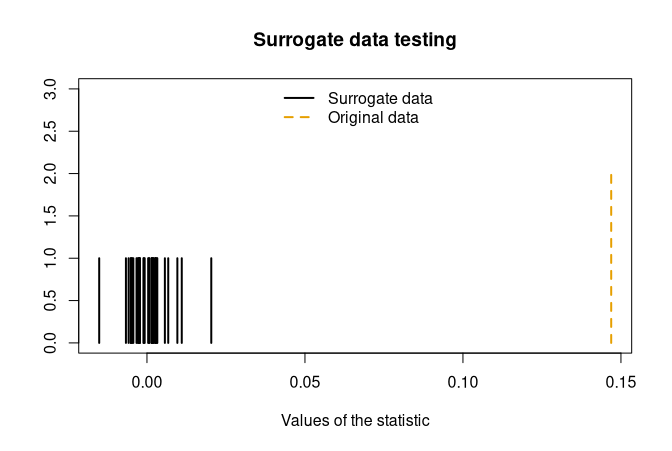## Other functionality included in the package

In this quickstart vignette we have only covered some of the main functions included in nonlinearTseries. Other interesting functions included in this package are enumerated below. The main reason for not including them in this quickstart guide is that these functions are quite simple to use.

• rqa: performs Recurrence Quantification Analysis.
• dfa: performs Detrended Fluctuation Analysis.
• nonLinearNoiseReduction: denoises a given time series using phase space techniques.
• poincareMap: computes a Poincare map of the trajectories in phase space.
• spaceTimePlot : shows the space time separation plot: broadly-used method of detecting non-stationarity and temporal correlations in time series.